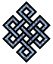#fail2ban bad ip database: ip 187.51.24.194

### | ip database | live view | stats | report | help | api key:

 ip: 187.51.24.194 hostname: 187-51-24-194.customer.tdatabrasil.net.br country:[BR] Brazil first reported: 03.10.2018 17:51.09 GMT+0200 last reported: 15.01.2019 21:19.01 GMT+0200 time period: 104d 04h 27m 52s total reports: 8 reported by: 2 host(s) filter(s): ssh (5) ssh (3) tor exit node no badips.com db Lookup## port scan of '187.51.24.194':

[-hide]
```# Nmap 6.40 scan initiated Wed Oct  3 17:52:03 2018 as: /usr/bin/nmap -sU -sS -O 187.51.24.194
Nmap scan report for 187-51-24-194.customer.tdatabrasil.net.br (187.51.24.194)
Host is up (0.23s latency).
Not shown: 1000 open|filtered ports, 990 filtered ports
PORT      STATE  SERVICE
1234/tcp  closed hotline
1236/tcp  closed bvcontrol
1244/tcp  closed isbconference1
1723/tcp  open   pptp
2121/tcp  closed ccproxy-ftp
2222/tcp  open   EtherNet/IP-1
50002/tcp open   iiimsf
50003/tcp open   unknown
60443/tcp open   unknown
No exact OS matches for host (If you know what OS is running on it, see http://nmap.org/submit/ ).
TCP/IP fingerprint:
OS:SCAN(V=6.40%E=4%D=10/3%OT=1723%CT=1234%CU=%PV=N%G=Y%TM=5BB4E6F5%P=x86_64
OS:-pc-linux-gnu)SEQ(SP=C8%GCD=1%ISR=CB%TI=Z%CI=RI%TS=A)OPS(O1=M5B4ST11NW2%
OS:O2=M5B4ST11NW2%O3=M5B4NNT11NW2%O4=M5B4ST11NW2%O5=M5B4ST11NW2%O6=M5B4ST11
OS:)WIN(W1=16A0%W2=16A0%W3=16A0%W4=16A0%W5=16A0%W6=16A0)ECN(R=Y%DF=Y%TG=40%
OS:W=16D0%O=M5B4NNSNW2%CC=N%Q=)T1(R=Y%DF=Y%TG=40%S=O%A=S+%F=AS%RD=0%Q=)T2(R
OS:=N)T3(R=Y%DF=Y%TG=40%W=16A0%S=O%A=S+%F=AS%O=M5B4ST11NW2%RD=0%Q=)T4(R=Y%D
OS:F=Y%TG=40%W=0%S=A%A=Z%F=R%O=%RD=0%Q=)T5(R=Y%DF=Y%TG=80%W=0%S=Z%A=S+%F=AR
OS:%O=%RD=0%Q=)T6(R=N)T7(R=Y%DF=Y%TG=40%W=0%S=Z%A=S+%F=AR%O=%RD=0%Q=)U1(R=N
OS:)IE(R=Y%DFI=N%TG=40%CD=S)

OS detection performed. Please report any incorrect results at http://nmap.org/submit/ .
# Nmap done at Wed Oct  3 17:57:41 2018 -- 1 IP address (1 host up) scanned in 339.67 seconds
```
```Σ = 52 | Δt = 0.0064330101013184s
```